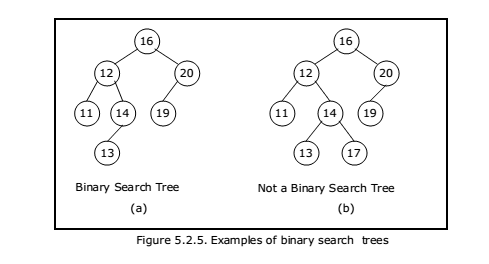# Concept Binary Search Tree A+ A  A-

A binary search tree is a binary tree. It may be empty. If it is not empty then it satisfies the following properties:

1.  Every element has a key and no two elements have the same key.
2. The keys in the left subtree are smaller than the key in the root.
3. The keys in the right subtree are larger than the key in the root.
4. The left and right subtrees are also binary search trees.

Figure 5.2.5(a) is a binary search tree, whereas figure 5.2.5(b) is not a binary search tree.